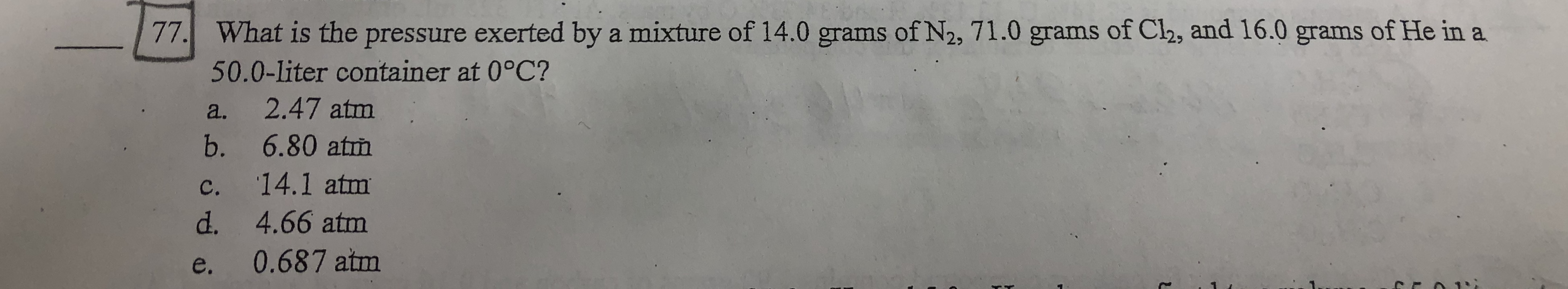What is the pressure exerted by a mixture of 14.0 grams of N2, 71.0 grams of Cl2, and 16.0 grams of He in a50.0-liter container at 0°C?a. 2.47 atmb. 6.80 atmc. 14.1 atmd. 4.66 atme. 0.687 atm77.

Questionhelp_outlineImage TranscriptioncloseWhat is the pressure exerted by a mixture of 14.0 grams of N2, 71.0 grams of Cl2, and 16.0 grams of He in a 50.0-liter container at 0°C? a. 2.47 atm b. 6.80 atm c. 14.1 atm d. 4.66 atm e. 0.687 atm 77. fullscreen
Step 1

Calculate the total moles of the given mixture,

Step 2

Use the ideal gas equation to calculate the pressure,

Step 3

Substitute the known, given and calculat...

Want to see the full answer?

See Solution

Want to see this answer and more?

Our solutions are written by experts, many with advanced degrees, and available 24/7

See Solution
Tagged in

Gas laws